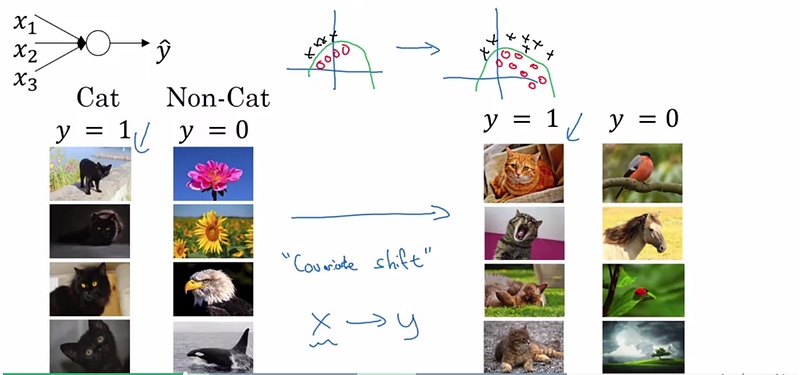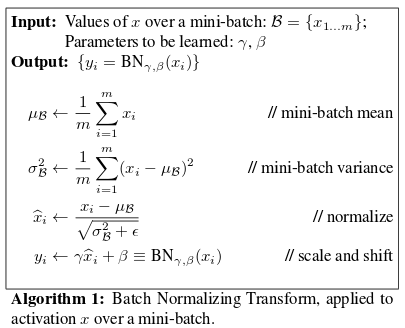# Batch Normalization in Neural Networkscomments

By Firdaouss Doukkali, Machine Learning Engineer

This article explains batch normalization in a simple way. I wrote this article after what I learned from Fast.ai and deeplearning.ai. I will start with why we need it, how it works, then how to include it in pre-trained networks such as VGG.

Why do we use batch normalization?

We normalize the input layer by adjusting and scaling the activations. For example, when we have features from 0 to 1 and some from 1 to 1000, we should normalize them to speed up learning. If the input layer is benefiting from it, why not do the same thing also for the values in the hidden layers, that are changing all the time, and get 10 times or more improvement in the training speed.

Batch normalization reduces the amount by what the hidden unit values shift around (covariance shift). To explain covariance shift, let’s have a deep network on cat detection. We train our data on only black cats’ images. So, if we now try to apply this network to data with colored cats, it is obvious; we’re not going to do well. The training set and the prediction set are both cats’ images but they differ a little bit. In other words, if an algorithm learned some X to Y mapping, and if the distribution of X changes, then we might need to retrain the learning algorithm by trying to align the distribution of X with the distribution of Y. ( Deeplearning.ai: Why Does Batch Norm Work? (C2W3L06))

Also, batch normalization allows each layer of a network to learn by itself a little bit more independently of other layers.Deeplearning.ai: Why Does Batch Norm Work? (C2W3L06)

• We can use higher learning rates because batch normalization makes sure that there’s no activation that’s gone really high or really low. And by that, things that previously couldn’t get to train, it will start to train.
• It reduces overfitting because it has a slight regularization effects. Similar to dropout, it adds some noise to each hidden layer’s activations. Therefore, if we use batch normalization, we will use less dropout, which is a good thing because we are not going to lose a lot of information. However, we should not depend only on batch normalization for regularization; we should better use it together with dropout.

How does batch normalization work?

To increase the stability of a neural network, batch normalization normalizes the output of a previous activation layer by subtracting the batch mean and dividing by the batch standard deviation.

However, after this shift/scale of activation outputs by some randomly initialized parameters, the weights in the next layer are no longer optimal. SGD ( Stochastic gradient descent) undoes this normalization if it’s a way for it to minimize the loss function.

Consequently, batch normalization adds two trainable parameters to each layer, so the normalized output is multiplied by a “standard deviation” parameter (gamma) and add a “mean” parameter (beta). In other words, batch normalization lets SGD do the denormalization by changing only these two weights for each activation, instead of losing the stability of the network by changing all the weights.From the original batch-norm paper

Batch normalization and pre-trained networks like VGG:

VGG doesn’t have a batch norm layer in it because batch normalization didn’t exist before VGG. If we train it with it from the start, the pre-trained weight will benefit from the normalization of the activations. So adding a batch norm layer actually improves ImageNet, which is cool. You can add it to dense layers, and also to convolutional layers.

If we insert a batch norm in a pre-trained network, it will change the pre-trained weights, because it will subtract the mean and divide by the standard deviation for the activation layers and we don’t want that to happen because we need those pre-trained weights to stay the same. So, what we need to do is to insert a batch norm layer and figure out gamma and beta in order to undo the outputs change.

To summarize everything, you can think about batch normalization as doing preprocessing at every layer of the network.

References:

Bio: Firdaouss Doukkali is a Machine Learning Engineer and Chief Unicorn Scientist. Global Shaper at World Economic Forum. English, French, German, Arabic, and Japanese speaker.

Original. Reposted with permission.

Related: